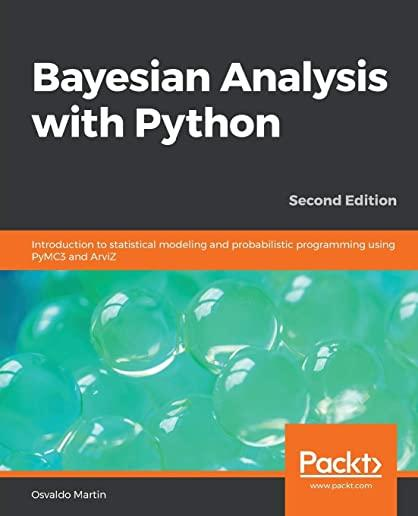### Bayesian Analysis with Python - Second Edition### Huge savings for students

Each student receives a 50% discount off of most books in the HSG Book Store. During class, please ask the instructor about purchase details.
 List Price: \$44.99 Price: \$22.50 You Save: \$22.50
3

Bayesian modeling with PyMC3 and exploratory analysis of Bayesian models with ArviZ

Key Features

• A step-by-step guide to conduct Bayesian data analyses using PyMC3 and ArviZ
• A modern, practical and computational approach to Bayesian statistical modeling
• A tutorial for Bayesian analysis and best practices with the help of sample problems and practice exercises.

Book Description

The second edition of Bayesian Analysis with Python is an introduction to the main concepts of applied Bayesian inference and its practical implementation in Python using PyMC3, a state-of-the-art probabilistic programming library, and ArviZ, a new library for exploratory analysis of Bayesian models.

The main concepts of Bayesian statistics are covered using a practical and computational approach. Synthetic and real data sets are used to introduce several types of models, such as generalized linear models for regression and classification, mixture models, hierarchical models, and Gaussian processes, among others.

By the end of the book, you will have a working knowledge of probabilistic modeling and you will be able to design and implement Bayesian models for your own data science problems. After reading the book you will be better prepared to delve into more advanced material or specialized statistical modeling if you need to.

What you will learn

• Build probabilistic models using the Python library PyMC3
• Analyze probabilistic models with the help of ArviZ
• Acquire the skills required to sanity check models and modify them if necessary
• Understand the advantages and caveats of hierarchical models
• Find out how different models can be used to answer different data analysis questions
• Compare models and choose between alternative ones
• Discover how different models are unified from a probabilistic perspective
• Think probabilistically and benefit from the flexibility of the Bayesian framework

Who this book is for

If you are a student, data scientist, researcher, or a developer looking to get started with Bayesian data analysis and probabilistic programming, this book is for you. The book is introductory so no previous statistical knowledge is required, although some experience in using Python and NumPy is expected.

Packt Publishing